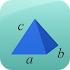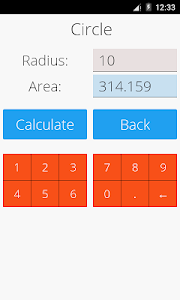# Area and Volume Calculator v21.5

Free

### Area and Volume Calculator / Screenshots### Area and Volume Calculator / Description

This free app is a geometric calculator which is able to calculate area and volume.

Area Calculator: You are able to calculate the area for the most important geometric figures. You can calculate the area of circle ellipse rectangle square trapezoid triangle parallelogram rhombus sector and triangle.

Volume Calculator: You are able to calculate the volume for the most important geometric objects. You can calculate the volume of cone cube cuboid cylinder sphere square pyramid and tetrahedron.

Best math tool for school and college! If you are a student it will help you to learn geometry!